Share

# Frank solutions for Class 10 Maths chapter 15 - Similarity

#### Frank Frank Class 10 Mathematics Part 2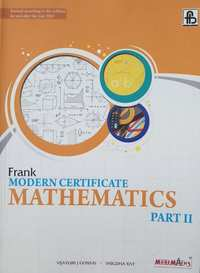## Chapter 15: Similarity

Exercise 15.1Exercise 15.2

#### Frank solutions for Class 10 Maths Chapter 15 Exercise Exercise 15.1 [Page 0]

In Δ ABC , DE is parallel to BC and "AD"/"DB" = 2/7 IF AC = 5 .6 , find AE.

Δ ABC ∼ Δ PQR. AD and PS are altitudes from A and P on sides BC and QR respectively. If AD : PS = 4 : 9 , find the ratio of the areas of Δ ABC and Δ PQR.

Δ ABC ~ Δ DEF. If BC = 3cm , EF=4cm and area of  Δ ABC = 54 cm2 ,  find area of  Δ DEF.

Δ ABC -  Δ XYZ. If area of  Δ ABC is 9cm2 and area of  Δ XYZ is 16cm2 and if BC= 2.1cm, find the length of YZ.

Prove that the area of  Δ BCE described on one side BC of a square ABCD is one half the area of the similar Δ ACF described on the diagonal AC.

If Δ ABC , MN || BC .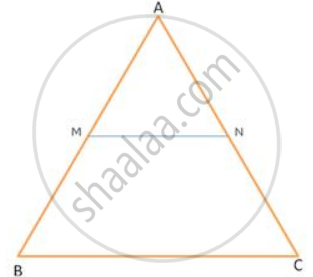If AN : AC= 5 : 8, find ar(Δ AMN) : ar(Δ ABC)

In Δ ABC , MN || BC .If "AB"/"AM" = 9/4 , find ("Ar" ("trapezium MBCN"))/("Ar" . (triangle "ABC"))

In Δ ABC , MN || BC .If BC = 14 cm and MN = 6 cm , find ("Ar" triangle "AMN")/("Ar" . ("trapezium MBCN"))

In  Δ ABC, DE || BC; DC and EB intersects at F. if "DE"/"BC" = 2/7 , find ("Ar" (triangle "FDE"))/("Ar" (triangle "FBC"))

Figure shows Δ PQR in which ST || QR and SR and QT intersect each other at M. If "PT"/"TR" = 5/3 find ("Ar" (triangle "MTS"))/("Ar" (triangle "MQR"))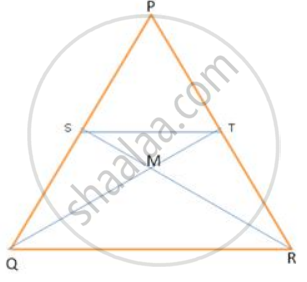Figure shows Δ KLM , P an T on KL and KM respectively such that∠ KLM =∠ KTP.

If "KL"/"KT" = 9/5 , find ("Ar" triangle "KLM")/("Ar" triangle "KTP").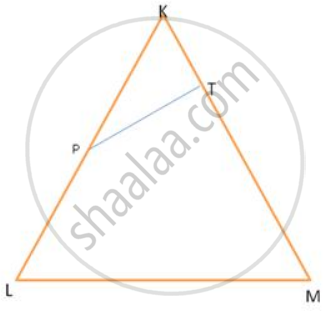In figure , DEF is a right -angled triangle with ∠ E = 90 °.FE is produced to G and GH is drawn perpendicular to DE = 8 cm , DH = 8 cm ,DH = 6 cm and HF = 4 cm , find ("Ar" triangle "DEF")/("Ar" triangle "GHF")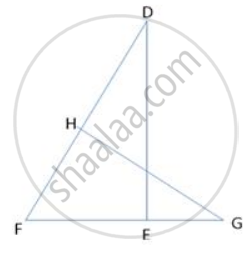In the figure , ABCD is a quadrilateral . F is a point on AD such that AF = 2.1 cm and FD = 4.9 cm . E and G are points on AC and AB respectively such that EF || CD and GE || BC . Find ("Ar" triangle "BCD")/("Ar" triangle "GEF")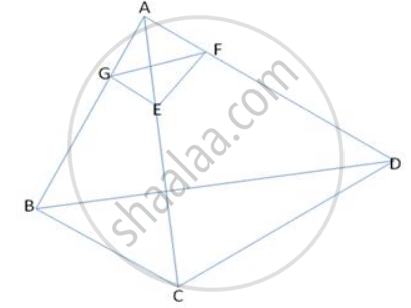In Δ PQR, MN is drawn parallel to QR. If PM = x, MQ = (x-2), PN = (x+2) and NR = (x-1), find the value of x.

Δ ABC is similar to Δ PQR. If AB = 6cm, BC = 9cm, PQ = 9cm and PR = 10.5cm, find the lengths of AC and QR.

ABCD and PQRS are similar figures. AB= 12cm, BC=x cm, CD= 15 cm, AD= 10 cm, PQ= 8 cm, QR = 5 cm, RS = m cm and PS = n cm .Find the values of x, m and n.

AD and BC are two straight lines intersecting at 0. CD and BA are perpendirulars from Band Con AD. If AB=6cm, CD =9cm, AD =20cm and BC=25cm, find the lengths of AO, BO, CO and DO.

In MBC, DE is drawn parallel to BC. If AD: DB=2:3, DE =6cm and AE =3.6cm, find BC and AC.

D and E are points on the sides AB and AC respectively of Δ ABC such that AB=5.6cm, AD= 1.4cm, AC=7 .2cm and AE = 1.5 cm, show that DE is parallel to BC

In  Δ ABC, D and E are points on the sides AB and AC respectively. If AD= 4cm, DB=4.Scm, AE=6.4cm and EC=7.2cm, find if DE is parallel to BC or not.

Δ ABC  ∼ Δ PQR such that AB= 1.5 cm and PQ=2. 1 cm. Find the ratio of areas of Δ ABC and  ΔPQR.

#### Frank solutions for Class 10 Maths Chapter 15 Exercise Exercise 15.2 [Page 0]

The dimensions of a buiIding are 50 m Iong, 40m wide and 70m high. A model of the same building is made with a scale factor of 1: 500. Find the dimensions of the model.

A ship is 400m laig and 100m wide. The length of its model is 20 cm. find the surface area of the deck of the model.

A model of a ship is made with a scale factor of 1 : 500. Find

The length of the ship, if the model length is 60 cm.

A model of a ship is made with a scale factor of 1 : 500. Find

The deck area of the model, if the deck area of the ship is 1500000 m2

A model of a ship is made with a scale factor of 1 : 500. Find

The volume of the ship, if the volume of its model is 200 cm3.

An aeroplane is 30m long and its model is l5 cm long. If the total outer surface area of the model is 150 cm2 , find the cost of painting the outer surface of the aeroplane at Rs. 120 per m2, if  5O m2 is left out for windows.

The length of a river in a map is 54cm. if lcm on the map represents 12500m on land, find the length of the river.

The scale of a map is 1 : 200000. A plot of land of area 20km2 is to be represented on the map. Find:

The number of kilometres on the ground represented by lcm or the map

The scale of a map is 1 : 200000. A plot of land of area 20km2 is to be represented on the map. Find:

The area in km2 that can be represented by 1 cm2

The scale of a map is 1 : 200000. A plot of land of area 20km2 is to be represented on the map. Find

The area on the map that represents the plot of land.

The actual area of an island is 1872km2 . On a map, this area is 117 cm2. if the length of the coastline is 44cm on the map, find the length of its actual coastline.

On a map drawn to a scale of 1 : 25000, a triangular plot of a land is marked as ABC with AB= 6cm, BC = 8cm and ∠ ABC = 90° . Calculate the actual length of AB in km and the actual area of the plot in km2

On a map drawn to a scale of 1 : 25000, a rectangular plot of land, ABCD is measured as AB= 12 cm and BC = 16cm. calculate the diagonal distance of the plot in km and the plot area in km2

## Chapter 15: Similarity

Exercise 15.1Exercise 15.2

#### Frank Frank Class 10 Mathematics Part 2## Frank solutions for Class 10 Mathematics chapter 15 - Similarity

Frank solutions for Class 10 Maths chapter 15 (Similarity) include all questions with solution and detail explanation. This will clear students doubts about any question and improve application skills while preparing for board exams. The detailed, step-by-step solutions will help you understand the concepts better and clear your confusions, if any. Shaalaa.com has the CISCE Frank Class 10 Mathematics Part 2 solutions in a manner that help students grasp basic concepts better and faster.

Further, we at Shaalaa.com provide such solutions so that students can prepare for written exams. Frank textbook solutions can be a core help for self-study and acts as a perfect self-help guidance for students.

Concepts covered in Class 10 Mathematics chapter 15 Similarity are Similarity of Triangles, Similarity Triangle Theorem, Axioms of Similarity of Triangles, Areas of Similar Triangles Are Proportional to the Squares on Corresponding Sides.

Using Frank Class 10 solutions Similarity exercise by students are an easy way to prepare for the exams, as they involve solutions arranged chapter-wise also page wise. The questions involved in Frank Solutions are important questions that can be asked in the final exam. Maximum students of CISCE Class 10 prefer Frank Textbook Solutions to score more in exam.

Get the free view of chapter 15 Similarity Class 10 extra questions for Maths and can use Shaalaa.com to keep it handy for your exam preparation

S